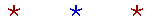S k i l l
i n
A R I T H M E T I C

Prologue 2

# THE MULTIPLICATION TABLE

 × 1 2 3 4 5 6 7 8 9 1 1 2 3 4 5 6 7 8 9 2 2 4 6 8 10 12 14 16 18 3 3 6 9 12 15 18 21 24 27 4 4 8 12 16 20 24 28 32 36 5 5 10 15 20 25 30 35 40 45 6 6 12 18 24 30 36 42 48 54 7 7 14 21 28 35 42 49 56 63 8 8 16 24 32 40 48 56 64 72 9 9 18 27 36 45 54 63 72 81

The student should practice the table out loud.  To practice the 8 table, for example, say,

"1 times 8 is 8.   2 times 8 is 16.   3 times 8 is 24."

And so on.  (Do not just say, "8,  16,  24, . . .")  Repeat this so that "3 times 8 is 24" begins to sound right.  It is not necessary to make an effort.  Repetition is all that is required to make an impression in the mind.

Practice each column until you can say the answer without thinking.

To see the answer, pass your mouse over the colored area.
To cover the answer again, click "Refresh" ("Reload").
Do the problem yourself first!

 6 × 4 = 24 5 × 4 = 20 7 × 4 = 28 7 × 7 = 49 9 × 8 = 72 9 × 3 = 27 7 × 5 = 35 9 × 9 = 81 6 × 3 = 18 9 × 5 = 45 8 × 4 = 32 6 × 8 = 48 3 × 9 = 27 4 × 6 = 24 4 × 5 = 20 5 × 7 = 35 4 × 7 = 28 3 × 6 = 18 4 × 3 = 12 7 × 8 = 56 8 × 7 = 56 8 × 3 = 24 6 × 7 = 42 8 × 9 = 72 5 × 8 = 40 5 × 6 = 30 3 × 4 = 12 4 × 8 = 32 5 × 5 = 25 5 × 9 = 45 8 × 6 = 48 8 × 5 = 40 9 × 6 = 54 6 × 9 = 54 7 × 9 = 63 6 × 5 = 30 9 × 7 = 63 9 × 4 = 36 6 × 6 = 36 3 × 8 = 24 3 × 7 = 21 7 × 3 = 21 8 × 8 = 64 3 × 5 = 15 5 × 3 = 15 7 × 6 = 42 4 × 9 = 36 4 × 4 = 16Here again are these elementary sums to practice.

 8 + 5 = 13 3 + 9 = 12 5 + 9 = 14 6 + 8 = 14 7 + 9 = 16 9 + 6 = 15 7 + 6 = 13 8 + 6 = 14 6 + 5 = 11 8 + 4 = 12 3 + 8 = 11 5 + 8 = 13 9 + 4 = 13 7 + 4 = 11 6 + 6 = 12 4 + 9 = 13 4 + 8 = 12 6 + 9 = 15 8 + 9 = 17 4 + 7 = 11 5 + 7 = 12 6 + 7 = 13 9 + 3 = 12 9 + 2 = 11 7 + 7 = 14 7 + 8 = 15 9 + 5 = 14 9 + 8 = 17 7 + 5 = 12 2 + 9 = 11 9 + 7 = 16 8 + 7 = 15 5 + 6 = 11 8 + 3 = 11 8 + 8 = 16 9 + 0 = 9 8 + 0 = 8 0 + 6 = 6 0 + 3 = 3 0 + 0 = 0

Lesson 1:  Powers of 10

Elementary Addition

www.proyectosalonhogar.com